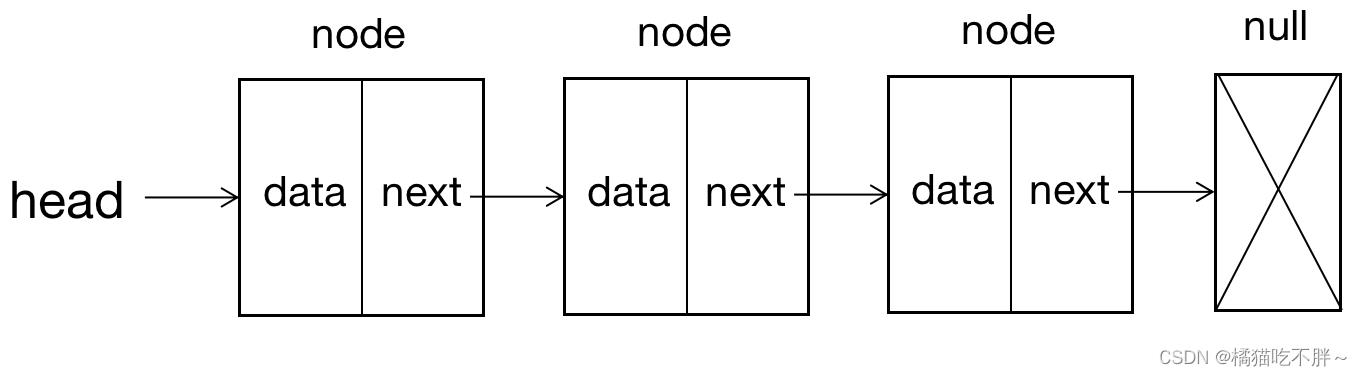﻿ JavaScript数据结构之链表各种操作详解 - 自由资讯

# JavaScript数据结构之链表各种操作详解

##### 目录
• 1 数组与链表的优缺点
• 2 什么是链表
• 3 封装链表结构
• 4 向链表尾部添加一个新的项
• 5 向链表某个位置插入一个新的项
• 6 获取对应位置的元素
• 7 获取元素在链表中的索引
• 8 修改某个位置的元素
• 9 从链表中删除某位置节点
• 10 全部代码

## 1 数组与链表的优缺点

• 数组的创建需要申请一段连续的内存空间（一整块的内存），并且大小是固定的，所以当当前数组不能满足容量需求时，就需要扩容（一般情况下会申请一个更大的数组，比如2倍，然后将原数组中的元素复制过去）。
• 在数组的开头或中间位置插入数据的成本很高，需要进行大量元素的位移。

• 内存空间不是必须连续的，可以充分利用计算机的内存，实现灵活的内存动态管理；
• 链表不必在创建时就确定大小，并且大小可以无限延伸下去；
• 链表在插入和删除数据时，时间复杂度可以达到O(1)，相对数组效率高很多；

• 链表访问任何一个元素的位置时，都需要从头开始访问，并且无法跳过第一个元素访问任何一个元素
• 链表无法通过下标直接访问元素，需要从头一个个访问，直到找到对应的元素

## 2 什么是链表## 3 封装链表结构

```function LinkedList() {
this.length = 0; // 初始链表长度为0
}
```

`LindedList`类内部有一个`ListNode`类，用于创建节点，创建节点时应该为节点传入数据`data`，并且该节点有指向下一个节点的指针。

```function LinkedList() {
this.length = 0; // 初始链表长度为0
function ListNode(data) {
this.data = data;
this.next = null;
}
}
```

## 4 向链表尾部添加一个新的项

• 当链表本身为空时，头节点就是新添加的节点
• 当链表不为空时，让链表最后一个节点指向新添加的节点

```LinkedList.prototype.append = function (data) { // data是新节点的值
let node = new ListNode(data); // 创建新的节点
if (this.length === 0) { // 如果链表为空，则新节点就是头节点
} else { // 如果链表不为空，新节点添加到链表尾部
let current = this.head; // 将current指向头节点
// 链表无法直接访问到最后的节点，只能通过一次次遍历来访问
while (current.next) { // 当达到最后一个节点时，循环结束
// 当下一个节点存在时，就让current指针移动到下一个节点上
current = current.next;
}
// 最后一个节点指向新节点
current.next = node;
}
this.length += 1; // 链表的长度+1
}
```

## 5 向链表某个位置插入一个新的项

• 当插入到第一个位置时，新节点变成了头节点，那么新节点要指向原来的头节点，属性`head`也应该变成新节点
• 当插入到其他位置时，首先通过循环找到该位置，同时保存上一个节点和下一个节点，然后将上一个节点指向新节点，新节点指向下一个节点

```// position为节点要插入的位置，data为节点的值
LinkedList.prototype.insert = function (position, data) {
// 对position进行越界判断，当该值小于0或者大于链表长度时，不能进行插入操作
if (position <= 0 || position > this.length) return false;
let node = new ListNode(data); // 创建新节点
if (position === 0) { // 如果节点要插入第一个位置
} else {
let previous = null; // 指向前一个位置
let current = this.head; // 指向下一个位置
let index = 1; // 记录循环的位置
// 循环结束，previous和current之间就是插入的节点
while (index < position) {
previous = current;
current = current.next;
index++;
}
previous.next = node; // 在正确的位置插入元素
node.next = current;
}
this.length += 1; // 长度加1
}```

## 6 获取对应位置的元素

```LinkedList.prototype.get = function (position) {
// 越界判断，如果位置小于0或者大于链表长度，不能获取到元素
if (position <= 0 || position > this.length) return null;
let index = 1; // 记录当前位置
let current = this.head; // current指向头节点
// 循环结束，current指向该位置上的节点
while (index < position) {
current = current.next;
index++;
}
return current.data;
}
```

## 7 获取元素在链表中的索引

```// 获取某个元素的位置
let index = 1; // 记录元素的位置
while (current) { // 循环遍历链表
if (current.data === data) { // 如果当前节点的值等于元素的值
return index; // 返回位置
} else { // 如果不等于，继续循环
current = current.next;
index++;
}
}
return -1; // 循环结束了，说明没找到
}
```

## 8 修改某个位置的元素

```// 修改某个位置的元素
LinkedList.prototype.update = function (position, data) {
// 越界判断
if (position <= 0 || position > this.length) return false;
let index = 1;
while (index < position) {
current = current.next;
index++;
}
current.data = data; // 修改数据
return true;
}
```

## 9 从链表中删除某位置节点

• 删除第一个位置上的节点时，要将第一个位置上的节点指向null，并且第二个位置上的节点成为头节点
• 删除其他位置上的节点，循环找到该位置，同时记录该节点上一个节，将上一个节点指向该位置的下一个节点

```LinkedList.prototype.removeAt = function (position) {
if (position <= 0 || position > this.length) return false; // 越界判断
if (position === 1) { // 如果删除第一个位置上的节点（头节点）
} else { // 删除其他位置的节点
let index = 1; // 记录当前位置
let previous = null;
while (index < position) {
previous = current;
current = current.next;
index++;
}
// 上一个节点指向当前元素的下一个节点
previous.next = current.next;
}
this.length--;
return current; // 返回被删除的节点
}
```

## 10 全部代码

```function LinkedList() {
this.length = 0; // 初始链表长度为0
function ListNode(data) { // 创建新节点类
this.data = data;
this.next = null;
}
// 添加元素
LinkedList.prototype.append = function (data) { // data是新节点的值
let node = new ListNode(data); // 创建新的节点
if (this.length === 0) { // 如果链表为空，则新节点就是头节点
} else { // 如果链表不为空，新节点添加到链表尾部
let current = this.head; // 将current指向头节点
// 链表无法直接访问到最后的节点，只能通过一次次遍历来访问
while (current.next) { // 当达到最后一个节点时，循环结束
// 当下一个节点存在时，就让current指针移动到下一个节点上
current = current.next;
}
// 最后一个节点指向新节点
current.next = node;
}
this.length += 1; // 链表的长度+1
}
// 插入元素
// position为节点要插入的位置，data为节点的值
LinkedList.prototype.insert = function (position, data) {
// 对position进行越界判断，当该值小于0或者大于链表长度时，不能进行插入操作
if (position <= 0 || position > this.length) return false;
let node = new ListNode(data); // 创建新节点
if (position === 0) { // 如果节点要插入第一个位置
} else {
let previous = null; // 指向前一个位置
let current = this.head; // 指向下一个位置
let index = 1; // 记录循环的位置
// 循环结束，previous和current之间就是插入的节点
while (index < position) {
previous = current;
current = current.next;
index++;
}
previous.next = node; // 在正确的位置插入元素
node.next = current;
}
this.length += 1; // 长度加1
}
// 获取某个位置上的元素
// 越界判断，如果位置小于0或者大于链表长度，不能获取到元素
if (position <= 0 || position > this.length) return null;
let index = 1; // 记录当前位置
let current = this.head; // current指向头节点
// 循环结束，current指向该位置上的节点
while (index < position) {
current = current.next;
index++;
}
return current.data;
}
// 获取某个元素的位置
let index = 1; // 记录元素的位置
while (current) { // 循环遍历链表
if (current.data === data) { // 如果当前节点的值等于元素的值
return index; // 返回位置
} else { // 如果不等于，继续循环
current = current.next;
index++;
}
}
return -1; // 循环结束了，说明没找到
}
// 修改某个位置的元素
LinkedList.prototype.update = function (position, data) {
// 越界判断
if (position <= 0 || position > this.length) return false;
let index = 1;
while (index < position) {
current = current.next;
index++;
}
current.data = data; // 修改数据
return true;
}
if (position <= 0 || position > this.length) return false; // 越界判断
if (position === 1) { // 如果删除第一个位置上的节点（头节点）
} else { // 删除其他位置的节点
let index = 1; // 记录当前位置
let previous = null;
while (index < position) {
previous = current;
current = current.next;
index++;
}
// 上一个节点指向当前元素的下一个节点
previous.next = current.next;
}
this.length--;
return current; // 返回被删除的节点
}
}```

﻿